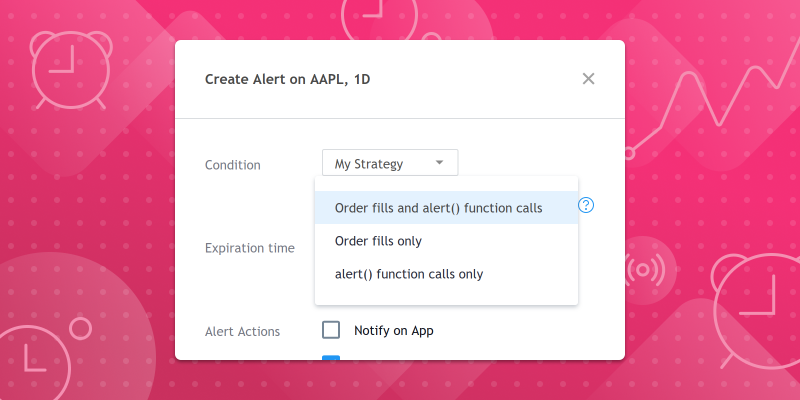January 25, 2021

1. 根据需要在脚本中包含尽可能多的`alert()`调用，并将每个调用都包含在定义触发条件的`if`块中。
2. 使用图表的“创建警报”对话框为脚本创建一个警报，然后选择一种警报类型，包括“ alert()函数调用”。

1）每根K线上都会触发脚本警报，并且消息中包含该K线的收盘价：

```//@version=4
study("Simple alert() example")
plot(close)
alert("Close = " + tostring(close), alert.freq_once_per_bar_close)```

2）检查三个指标的值：RSI，SMA和动量。如果任何指标超过指定水平（或价格），都会触发脚本警报，并且消息中将包含指标名称及其当前值。

```//@version=4
study("alert() with multiple indicators", overlay=true)
f_triggerSma()=>
_s  = sma(close, 14)
_co = crossover(close, _s)
_cu = crossunder(close, _s)
if _co
alert("Price (" + tostring(close) + ") crossing up SMA (" + tostring(_s) + ")", alert.freq_once_per_bar)
else if _cu
alert("Price (" + tostring(close) + ") crossing down SMA (" + tostring(_s) + ")", alert.freq_once_per_bar)
f_triggerRsi()=>
_r  = rsi(close, 7)
_co = crossover(_r, 70)
_cu = crossunder(_r, 30)
if _co
alert("RSI (" + tostring(_r) + ") crossing up 70 level", alert.freq_once_per_bar)
else if _cu
alert("RSI (" + tostring(_r) + ") crossing down 30 level", alert.freq_once_per_bar)
f_triggerMom()=>
_m  = mom(close, 14)
_co = crossover(_m, 0)
_cu = crossunder(_m, 0)
if _co
alert("Momentum (" + tostring(_m) + ")  crossing up 0 level", alert.freq_once_per_bar)
else if _cu
alert("Momentum (" + tostring(_m) + ")  crossing down 0 level", alert.freq_once_per_bar)

plot(sma(close, 14), "SMA")
f_triggerSma()
f_triggerRsi()
f_triggerMom()```

3）在此示例中，检查了一个RSI指标的值，但一次检查了五个商品。如果RSI穿过任何一个商品的指定水平，将触发脚本警报，并且消息将在穿过时包含该商品和指标值。

```//@version=4
study("alert() with multiple symbols")
f_triggerRsi(_ticker)=>
_r = rsi(close, 7)
_x = crossover(_r,70)
_y = crossunder(_r,30)
[_co, _cu] = security(_ticker, timeframe.period, [_x, _y])
_msg = _ticker + ", " + timeframe.period + ": "
if _co
_msg := _msg + "RSI (" + tostring(_r) + ") crossing up 70 level"
alert(_msg,  alert.freq_once_per_bar_close)
else if _cu
_msg := _msg + "RSI (" + tostring(_r) + ")  crossing down 30 level"
alert(_msg,  alert.freq_once_per_bar_close)

plot(rsi(close, 7), "RSI", color=#8E1599)
band1 = hline(70, "Upper Band", color=#C0C0C0)
band0 = hline(30, "Lower Band", color=#C0C0C0)
fill(band1, band0, color=#9915FF, transp=90, title="Background")

f_triggerRsi(syminfo.tickerid)
f_triggerRsi("NASDAQ:MSFT")
f_triggerRsi("FX:EURUSD")
f_triggerRsi("NASDAQ:TSLA")
f_triggerRsi("NASDAQ:PYPL")```